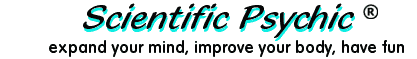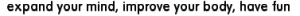Index# The Leaning Tower Problem - part 1

It is said that Galileo (1564-1642) dropped balls of various weights from the top of the Leaning Tower of Pisa to refute an Aristotelian belief that heavier objects fall faster than lighter objects.

If the balls were dropped from a height of 54 meters, how long did it take for the balls to hit the ground?

To solve this problem, it is necessary to know that the gravity of the earth accelerates objects at a rate of 9.8 meters per second squared and then use the laws of gravitation formulated by Isaac Newton (1642-1727).

The distance that an object travels under constant acceleration is given by the formula:

distance = 1/2 × acceleration × time2 + intial speed × time

The initial speed is zero because the balls are dropped and not thrown. Therefore, we can solve for time:

time2 = (2 × distance) / acceleration

Substituting distance = 54 meters and acceleration = 9.8 meters/second2, we get

time2 = (2 × 54 meters) / (9.8 meters/second2) = 11.0 seconds2

The square root gives:

time = 3.3 seconds